Chapter 18. Chemical Thermodynamics

# Measuring Entropy and Entropy Changes

Jessie A. Key

Learning Objectives

• To gain an understanding of methods of measuring entropy and entropy change.

As the temperature of a sample decreases, its kinetic energy decreases and, correspondingly, the number of microstates possible decreases. The third law of thermodynamics states: at absolute zero (0 K), the entropy of a pure, perfect crystal is zero. In other words, at absolute zero, there is only one microstate and according to Boltzmann’s equation: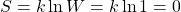Using this as a reference point, the entropy of a substance can be obtained by measuring the heat required to raise the temperature a given amount, using a reversible process. Reversible heating requires very slow and very small increases in heat.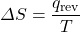Example 18.1

Determine the change in entropy (in J/K) of water when 425 kJ of heat is applied to it at 50°C. Assume the change is reversible and the temperature remains constant.

Solution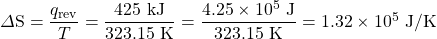# Standard Molar Entropy, S°

The standard molar entropy, S°, is the entropy of 1 mole of a substance in its standard state, at 1 atm of pressure. These values have been tabulated, and selected substances are listed in Table 18.1a to c “Standard Molar Entropies of Selected Substances at 298 K”.

Table 18.1a Standard Molar Entropies of Selected Gases at 298 K
Gas S°[J/(mol·K)]
He 126.2
H2 130.7
Ne 146.3
Ar 154.8
Kr 164.1
Xe 169.7
H2O 188.8
N2 191.6
O2 205.2
CO2 213.8
I2 260.7
Table 18.1b Standard Molar Entropies of Selected Liquids at 298 K
Liquid S°[J/(mol·K)]
H2O 70.0
CH3OH 126.8
Br2 152.2
CH3CH2OH 160.7
C6H6 173.4
CH3COCl 200.8
C6H12 (cyclohexane) 204.4
C8H16 (isooctane) 329.3
Table 18.1c Standard Molar Entropies of Selected Solids at 298 K
Solid S°[J/(mol·K)]
C (diamond) 2.4
C (graphite) 5.7
LiF 35.7
SiO2 (quartz) 41.5
Ca 41.6
Na 51.3
MgF2 57.2
K 64.7
NaCl 72.1
KCl 82.6
I2 116.1

Several trends emerge from standard molar entropy data:

• Larger, more complex molecules have higher standard molar enthalpy values than smaller or simpler molecules. There are more possible arrangements of atoms in space for larger, more complex molecules, increasing the number of possible microstates.
• Gases tend to have much larger standard molar enthalpies than liquids, and liquids tend to have larger values than solids, when comparing the same or similar substances.
• The standard molar entropy of any substance increases as the temperature increases. This can be seen in Figure 18.3 “Entropy vs. Temperature of a Single Substance.” Large jumps in entropy occur at the phase changes: solid to liquid and liquid to gas. These large increases occur due to sudden increased molecular mobility and larger available volumes associated with the phase changes.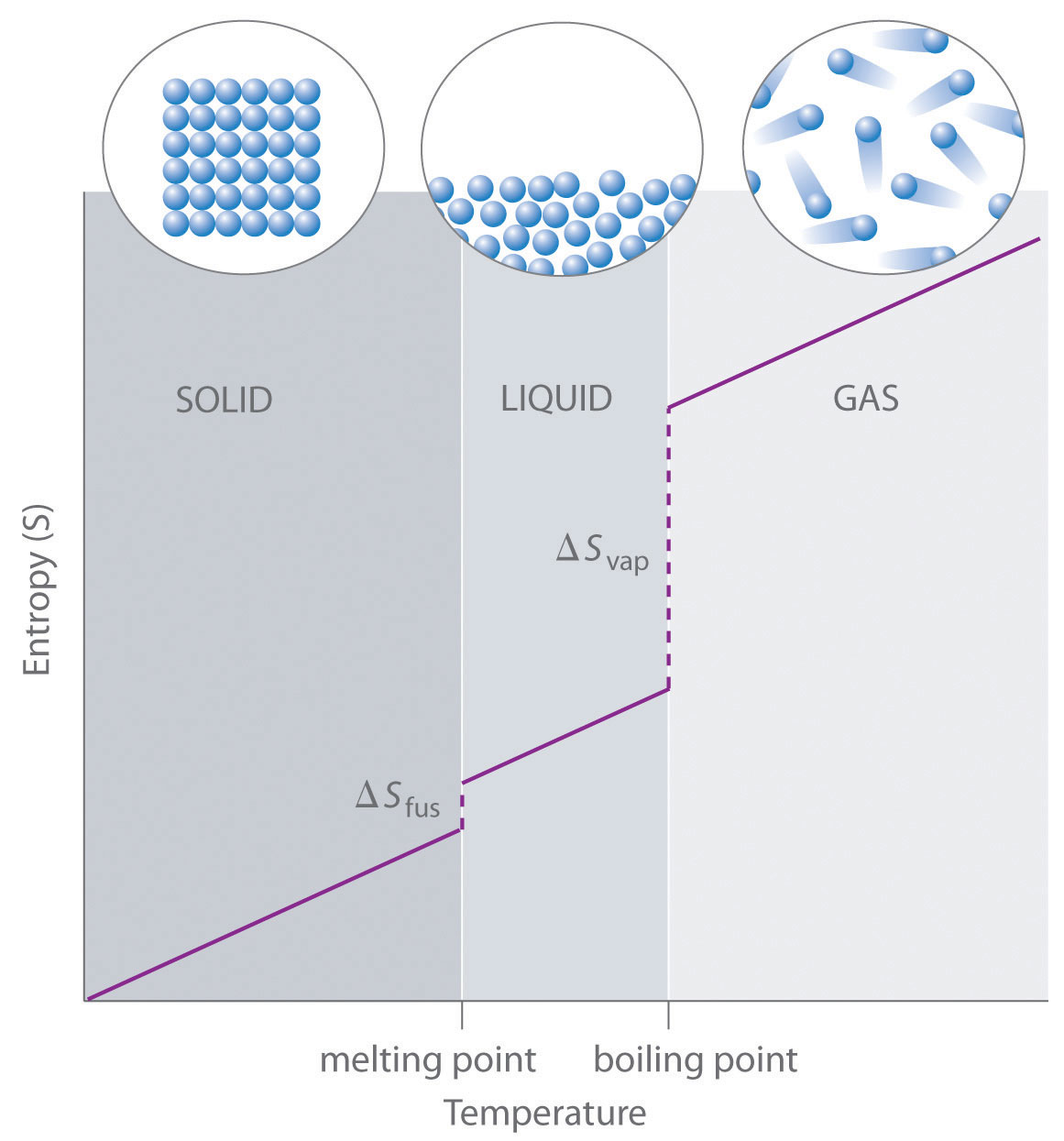Figure 18.3 “Entropy vs. Temperature of a Single Substance.” This is a generalized plot of entropy versus temperature for a single substance. (Source: UC Davis ChemWiki by University of California\CC-BY-SA-3.0)

# Standard Entropy Change of a Reaction, ΔS°

The entropy change of a reaction where the reactants and products are in their standard state can be determined using the following equation: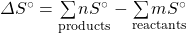where n and m are the coefficients found in the balanced chemical equation of the reaction.

Example 18.2

Determine the change in the standard entropy, ΔS°, for the synthesis of carbon dioxide from graphite and oxygen:

C(s) + O2(g) → CO2(g)

Solution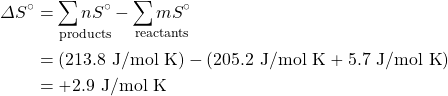# Entropy Changes in the Surroundings

The second law of thermodynamics states that a spontaneous reaction will result in an increase of entropy in the universe. The universe comprises both the system being examined and its surroundings.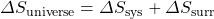Standard entropy change can also be calculated by the following: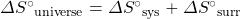The change in entropy of the surroundings is essentially just a measure of how much energy is being taken in or given off by the system. Under isothermal conditions, we can express the entropy change of the surroundings as: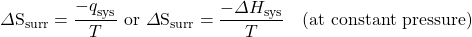Example 18.3

For the previous example, the change in the standard entropy, ΔS°, for the synthesis of carbon dioxide from graphite and oxygen, use the previously calculated ΔS°sys and standard enthalpy of formation values to determine S°surr and ΔS°universe.

Solution

First we should solve for the ΔH°sys using the standard enthalpies of formation values: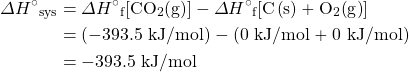Now we can convert this to the ΔS°surr: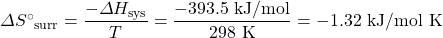Finally, solve for ΔS°universe: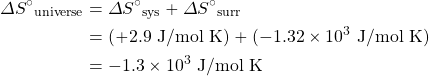Key Takeaways

• At absolute zero (0 K), the entropy of a pure, perfect crystal is zero.
• The entropy of a substance can be obtained by measuring the heat required to raise the temperature a given amount, using a reversible process.
• The standard molar entropy, S°, is the entropy of 1 mole of a substance in its standard state, at 1 atm of pressure.

1. These tables are based on a table from UC Davis ChemWiki by University of California\CC-BY-SA-3.0